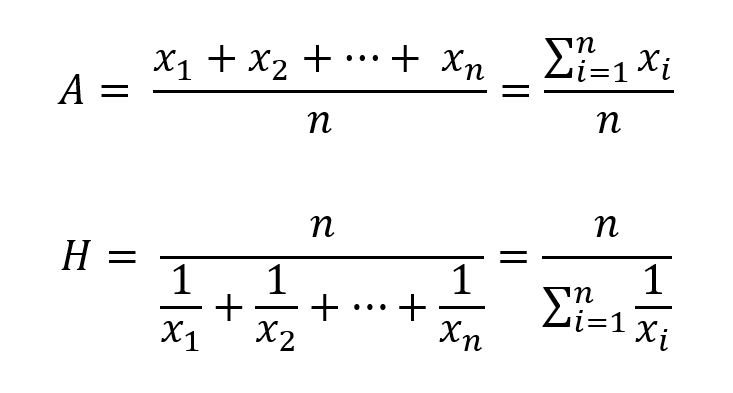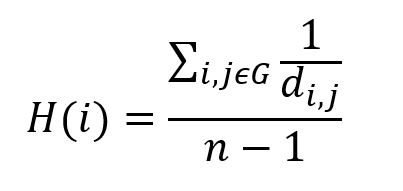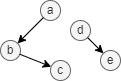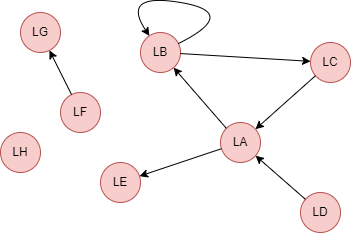# Change Nickname

Current Nickname:

Search
v4.0
v4.0

# Harmonic Centrality

## Overview

Harmonic Centrality algorithm is a variant of Closeness Centrality algorithm. Closeness centrality measures the average shortest distance from node to other nodes in its connected component but cannot describe the centrality of the node in the whole graph which is disconnected. The 'average shortest distance' proposed by the Harmonic Centrality algorithm calculates the sum of the reciprocals of these shortest distances, which allows it to handle the infinite values that appear in disconnected graph. Harmonic Centrality algorithm was first proposed by M. Marchiori and V. Latora in 2000, and then proposed by A. Dekker and Y. Rochat in 2005 and 2009.

The range of values of harmonic centrality is [0,1]; the larger the value, the closer the node is to the center.

Related materials of this algorithm:

## Basic Concept

### Shortest Distance

The shortest distance is the number of edges in the shortest path between two nodes. Please refer to chapter Closeness Centrality for more details.

### Harmonic Mean

Harmonic mean is a kind of mean, and unlike arithmetic mean, it is the reciprocal of the arithmetic mean of the reciprocals of the variables, so it is also called the reciprocal mean. The formula for calculating the arithmetic mean `A` and the harmonic mean `H` is as follows:A classic application of the harmonic mean is to travel the same distance at different speeds. Suppose there is a round trip with a speed of 30 km/h for the departure trip and 10 km/h for the return trip, what is the average speed for the entire trip?

Some people might use the arithmetic mean to calculate `A = (30+10)/2 = 20 km/h` without thinking, but the result is not very reasonable. The departure trip takes less time due to the higher speed, and the return trip takes more time, the speed for most of the entire trip is at 10 km/h, so the average speed should be closer to 10 km/h.

Assuming the one-way distance is `1`, the average speed which takes travel time into consideration is `2/(1/30+1/10) = 15 km/h`, which is the harmonic mean, and this is the true average speed, it is adjusted according to the time spent per distance.

### Harmonic Centrality

The harmonic centrality of a node is defined as the reciprocal of the harmonic mean of the shortest distances from the node to all other nodes in the graph:where `i` is the node to be calculated, `j` is the nodes in the graph other than `i`, `n-1` is the number of `j`, `d(i,j)` is the shortest distance between `i` and `j`, `d(i,j) = +∞` when there is no edge between `i` and `j` and `1/d(i,j) = 0`.The harmonic centrality of node `a` in the above graph is `(1 + 1/2 + 1/+∞ + 1/+∞) / 4 = 0.375`, and the harmonic centrality of node `b` is `(1/+∞ + 1/+∞ + 1/+∞ + 1) / 4 = 0.25`.

Harmonic Centrality algorithm consumes considerable computing resources because all the shortest paths from a node in the whole graph are to be calculated. You may perform sampling harmonic centrality calculation on GraphSet with more than 10,000 nodes, the suggested number of the samples is the logarithm based on 10 `log(number of nodes)`; user can configure whether to sample or not.

## Special Case

### Lonely Node, Disconnected Graph

Harmonic centrality of node considers nodes in the graph, including lonely nodes and nodes in different connected components.

### Self-loop Edge

It's the shortest distance between nodes that harmonic centrality calculates, since self-loop edge doesn't constitute the shortest path, it does not participate in the calculation.

### Directed Edge

When harmonic centrality is calculated without specifying the direction of edge, the direction of edge is ignored, and all nodes in the graph are involved in the calculation.

## Results and Statistics

Take the graph of 8 nodes below as an example, run the Harmonic Centrality algorithm against all nodes:Algorithm results: Calculate centrality for each node while ignoring edge direction , return `_id`, `centrality` or `_uuid`, `centrality` according to the execution method

_uuid _id centrality
1 LA 0.5714286
2 LB 0.4285714
3 LC 0.4285714
4 LD 0.3571429
5 LE 0.3571429
6 LF 0.1428571
7 LG 0.1428571
8 LH 0.0000000

Algorithm statistics: N/A

## Command and Configuration

• Command: `algo(harmonic_centrality)`
• Configurations for the parameter `params()`:
Name
Type
Default
Specification
Description
ids / uuids []`_id` / []`_uuid` / / IDs or UUIDs of nodes to be calculated, settings of `sample_size` is invalid when certain nodes are specified here; all nodes to be calculated if not set, this is when the settings of `sample_size` is valid
direction string / in/out, case insensitive Direction of edges in path; direction ignored if not set
sample_size int -1 -1, -2 or (0,Number of nodes] Number of nodes to be sampled, -1 means to sample `log(<number of nodes in the whole graph)` nodes, no sampling is applied and use all nodes to calculate precisely if sets to -2 or not set
limit int -1 >=-1 Number of results to return; return all results if sets to -1 or not set
order string / ASC/DESC, case insensitive To sort the returned results; no sorting is applied if not set

Example: Calculate harmonic centrality of nodes UUID = 1,2,3,4

``````algo(harmonic_centrality).params({
uuids: [1,2,3,4]
}).stream() as centrality
return centrality
``````

Example: Sampling calculate outbound harmonic centrality of all nodes, return 5 results

``````algo(harmonic_centrality).params({
limit: 5,
direction: "out",
sample_size: -1
}) as out
return out
``````

## Algorithm Execution

#### 1. File Writeback

Configuration Data in Each Row
filename `_id`,`centrality`

Example: Calculate harmonic centrality of all nodes, write the algorithm results back to file named centrality

``````algo(harmonic_centrality).params().write({
file:{
filename: "centrality"
}
})
``````

#### 2. Property Writeback

Configuration Writeback Content Type Data Type
property `centrality` Node property `float`

Example: Calculate harmonic centrality of all nodes, write the centrality back to node property named hc

``````algo(harmonic_centrality).params().write({
db:{
property: "hc"
}
})
``````

#### 3. Statistics Writeback

This algorithm has no statistics.

### Direct Return

Alias Ordinal Type
Description
Column Name
0 []perNode Node and its harmonic centrality `_uuid`, `centrality`

Example: Calculate harmonic centrality of all nodes, define algorithm results as alias named results, and return the 3 results with the highest centrality

``````algo(harmonic_centrality).params({
order: "desc",
limit: 3
}) as results
return results
``````

### Streaming Return

Alias Ordinal Type
Description
Column Name
0 []perNode Node and its harmonic centrality `_uuid`, `centrality`

Example: Calculate harmonic centrality of all nodes, define algorithm results as alias named results, and return the results with centrality equals to 0

``````algo(harmonic_centrality).params().stream() as results
where results.centrality == 0
return results
``````

### Real-time Statistics

This algorithm has no statistics.